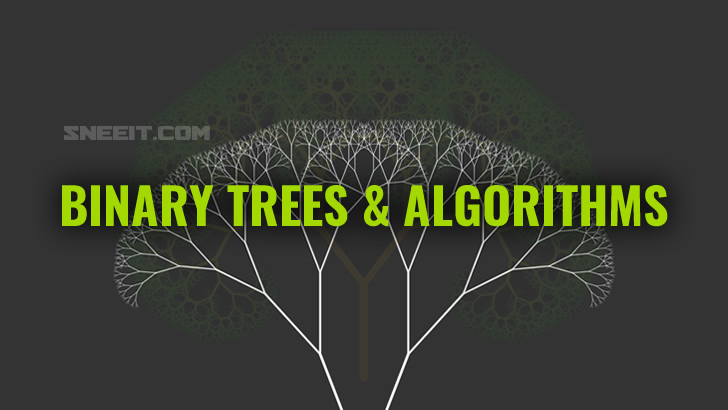# Binary Trees and Algorithms

Binary Tree is a very popular data structure. A tree has nodes and each node may have child nodes. In a binary tree, the total child nodesBinary Tree is a very popular data structure. A tree has nodes and each node may have child nodes. In a binary tree, the total child nodes that a node could have are 2, which are usually referred to as the left child and the right child.

By completing this course, you will able to:

• Understand the concept of a binary tree
• Be familiar with some basic algorithms for binary trees
• Be familiar with some variants of binary trees and their problems
Start to learn the lessons